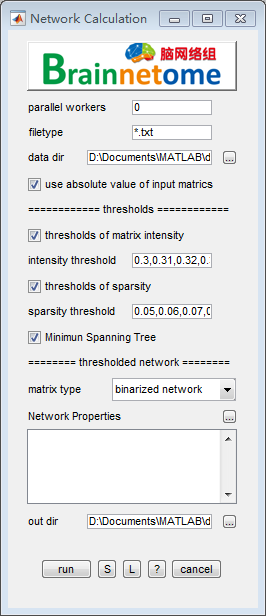Network Calculation¶• parallel workers: number of workers used for parallel computing.
• filetype: files in the filetype will be searched in input directories.
• data dir: directory where all `*.txt` correlation matrix results are stored.
• use absolute value of input matrics:
• raw value: use raw value to construct binary matrix.
• absolute value: use absolute value to construct binary matrix.
• intensity threshold: vector of thresholds for matrix intensity. e.g. correlation coefficient
• sparsity threshold: a vector of sparsity threshold, for each element, threshold the input matrix using the fraction of the matrix’s largest number of connection n*(n+1)/2;
• minimum spanning tree: a process to avoid unconnected network. To label the backbone of the network’s nodes.
• matrix type: binarized and weighted network. The binarized networks comes from thresholded input matrics, while the weighted network comes from a dot product operation of binarized network and the original network.
• Network properies: a panel to select network properties. In the option panel, (*) means the calculation of the property is slow.
• out dir: output directory for saving results.
• zero value in clustering coefficient: if the network is connected in a way (e.g. Hamilton path) that neighbour nodes are not connected; or the current node has only one neighbour node.
• Inf in small worldness: The small-worldness is calculated as real-network / random-network, and in real and random case, small-worldness is calculated as clustering coefficient/shortest-path length. If the mean clustering coefficient of the random-network is zero, then the small-worldness of random-network is zero, and it will cause the real-network / random-network to be Inf(any non-zero divided by 0). The solution is set smaller thresholds that avaliable for all subjects.
• Buttons:
• S: Save parameters of the current panel to a `*.mat` file. The `*.mat` can be further loaded for the panel or be used in a script processing.
• L: Load parameters from `*.mat` for the current panel.
• ?: Help information.
• Reference:
1. Rubinov M, Sporns O. Complex network measures of brain connectivity: uses and interpretations. Neuroimage 2010; 52(3): 1059-69.